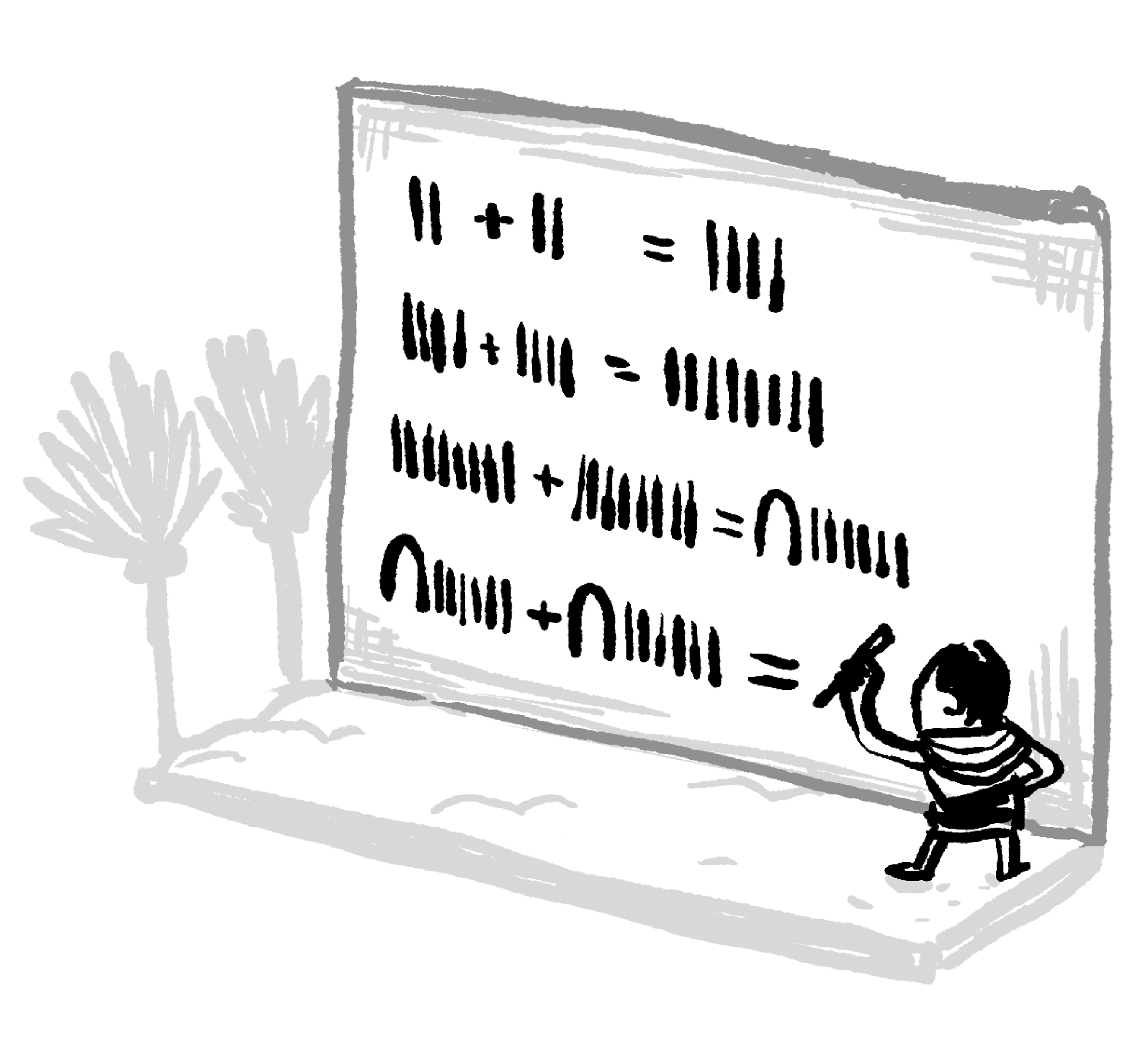Back

## Consecutive Sum

Every positive integer is the sum of powers of two, with no power repeating. For example,

\begin{aligned} 13 & = 1 + 4 + 8 \\ & = 2^0 + 2^2 + 2^3. \end{aligned}

Is there a quick way to tell whether an integer is the sum of consecutive powers of two, though? Keep reading to find out, or jump ahead to the challenge if you think you already know.

Ancient Egyptians broke down numbers into powers of two as part of their multiplication system. To find powers of two, they just needed to add repeatedly. The fewer powers of two one of the numbers was made of, the better, since that would mean less work to multiply the numbers.If both numbers were the sum of many powers of two, though, there would be more work for the scribe to do. Numbers that were the sum of consecutive powers of two meant a lot of work. One example is $30 \times 124,$ since $30 = 2 + 4 + 8 + 16$ and $124 = 4 + 8 + 16 + 32 + 64.$

Let's look at a sum of consecutive powers of two that starts with $2^0 = 1,$ and call it $s:$

$s = 1 + 2 + 4 + 8 + 16 + 32.$

Now here's $s,$ doubled:

$2s = 2 + 4 + 8 + 16 + 32 + 64.$

Subtracting $2s - s$ then leaves us with $s,$ but in a much simpler form than what we started with:

That leaves us with

\begin{aligned} s &= 64 - 1 \\ & = 63. \end{aligned}

Any time we do this with a sum of consecutive powers of two (starting with one), the middle terms cancel out.

What kind of number will a sum like this always be?

# Today's Challenge

Every positive integer can be written as the sum of distinct powers of two. For example,

\begin{aligned} 13 & = 1 + 4 + 8 \\ & = 2^0 + 2^2 + 2^3. \end{aligned}

Which of the following integers is the sum of consecutive powers of two?We are sunsetting our community features by July 2, 2021. Learn more here.
×

Problem Loading...

Note Loading...

Set Loading...